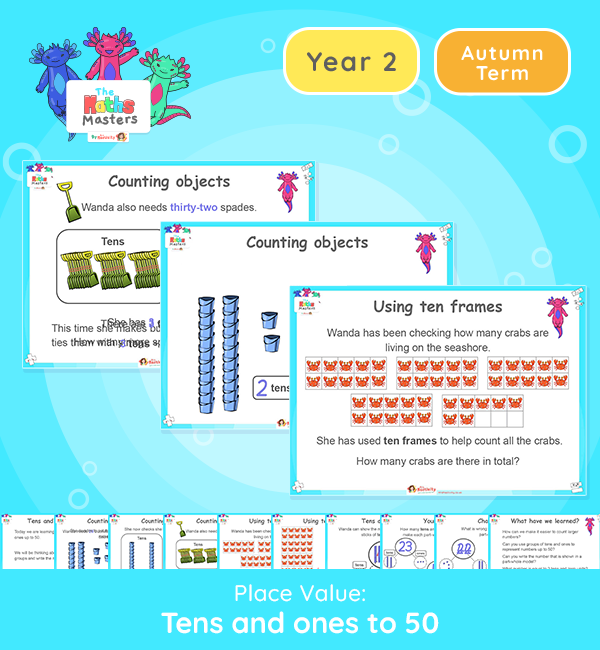Year 2 | Tens and Ones to 50 Lesson PresentationYear 2 White Rose Maths Place Value Resources

This is the second lesson on tens and ones. It shows the use of base ten and other groups of tens and ones to represent numbers within 50. It recaps Year 1 objectives at the start of Year 2.

Aligned with the White Rose Maths scheme of work, this Year 2 | Tens and Ones to 50 Presentation is fully editable, and is designed for the Year 2 maths curriculum covering the following maths objectives for the autumn term:

Topic/Block: Place Value

White Rose Maths Small Steps: Tens and ones within 50.

NC Links: Recognise the place value of each digit in a two digit number (tens, ones). • Identify, represent and estimate numbers using different representations including the number line.

Ready-to-progress criteria: Year 1 Conceptual Prerequisites: Know that 10 ones are equivalent to 1 ten.

2NPV-1 Recognise the value of each digit in two-digit numbers, and compose and decompose two-digit numbers using standard and non-standard partitioning.

TAF Statements: Working towards – Partition a two-digit number into tens and ones and demonstrate an understanding of place value, though they may use structured resources to support them.

Working at – Partition two digit numbers into different combinations of tens and ones, explaining their thinking verbally, in pictures or using apparatus.

There are teacher prompts and notes included in the “note” section of each slide.

Aligned with the order of teaching of the White Rose Maths scheme of work, use this to help your class get to grips with each mathematical concept. This lesson presentation also includes varied fluency activities, problem solving, and mathematical discussion questions too.

Explore our other year 2 place value resources.